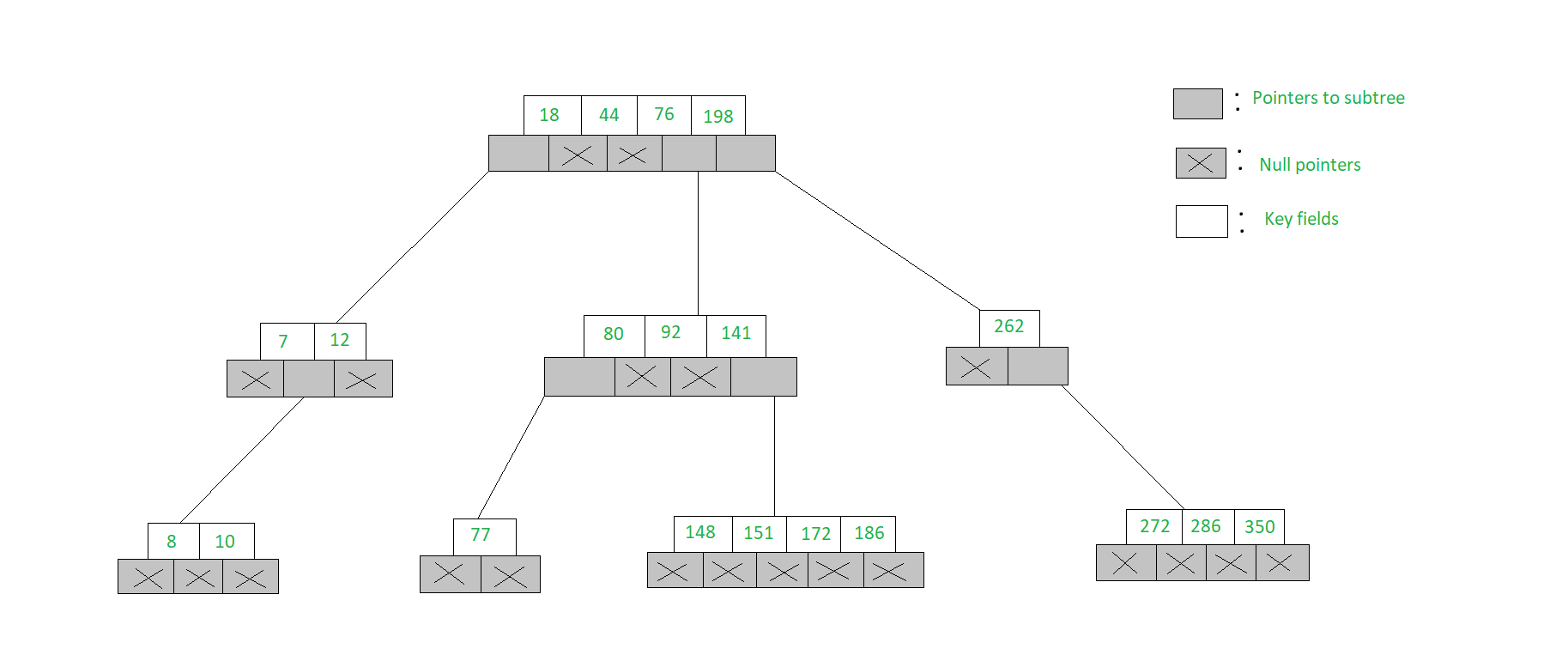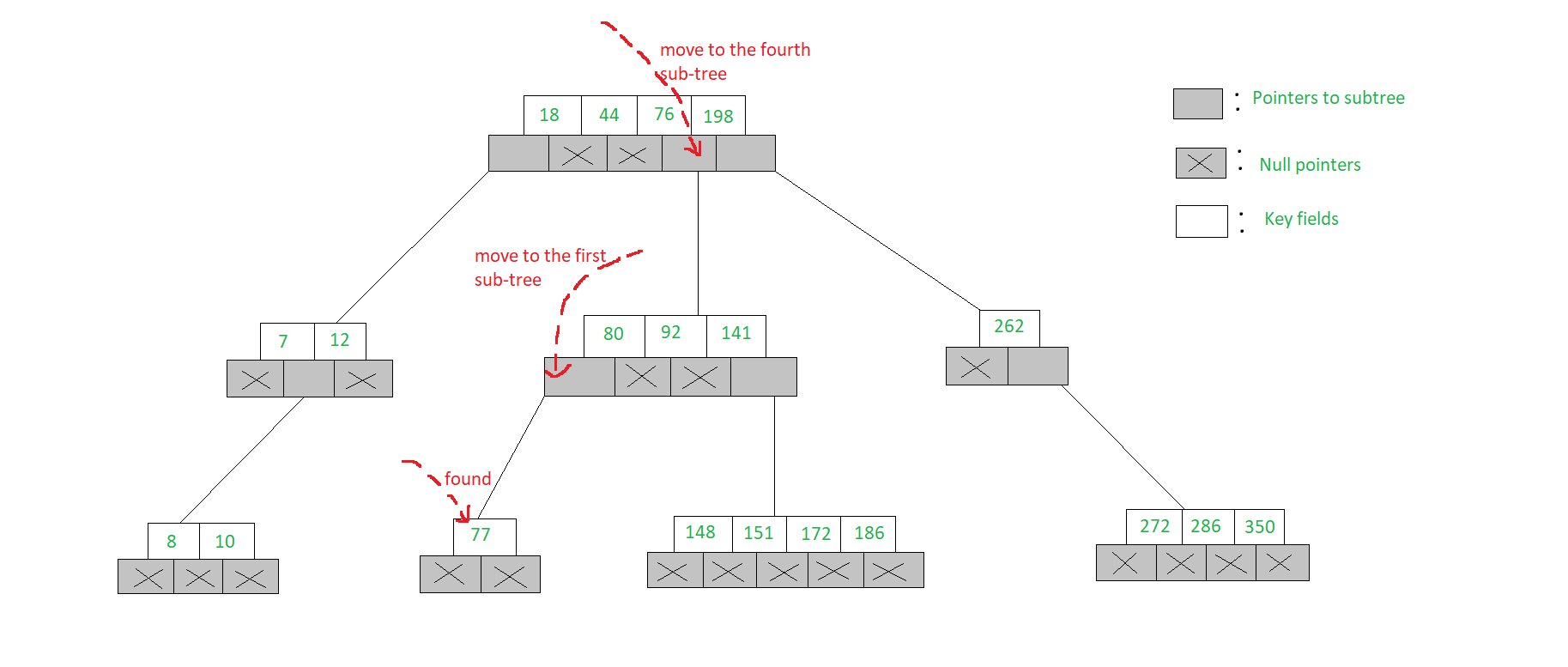# m-WAY Search Trees | Set-1 ( Searching )

The m-way search trees are multi-way trees which are generalised versions of binary trees where each node contains multiple elements. In an m-Way tree of order m, each node contains a maximum of m – 1 elements and m children.

The goal of m-Way search tree of height h calls for O(h) no. of accesses for an insert/delete/retrieval operation. Hence, it ensures that the height h is close to log_m(n + 1).

The number of elements in an m-Way search tree of height h ranges from a minimum of h to a maximum of.

An m-Way search tree of n elements ranges from a minimum height of log_m(n+1) to a maximum of n

An example of a 5-Way search tree is shown in the figure below. Observe how each node has at most 5 child nodes & therefore has at most 4 keys contained in it.The structure of a node of an m-Way tree is given below:

 `struct` `node { ` `    ``int` `count; ` `    ``int` `value[MAX + 1]; ` `    ``struct` `node* child[MAX + 1]; ` `}; `

• Here, count represents the number of children that a particular node has
• The values of a node stored in the array value
• The addresses of child nodes are stored in the child array
• The MAX macro signifies the maximum number of values that a particular node can contain

Searching in an m-Way search tree:

• Searching for a key in an m-Way search tree is similar to that of binary search tree
• To search for 77 in the 5-Way search tree, shown in the figure, we begin at the root & as 77> 76> 44> 18, move to the fourth sub-tree
• In the root node of the fourth sub-tree, 77< 80 & therefore we move to the first sub-tree of the node. Since 77 is available in the only node of this sub-tree, we claim 77 was successfully searched`// Searches value in the node ` `struct` `node* search(``int` `val, ` `                    ``struct` `node* root, ` `                    ``int``* pos) ` `{ ` ` `  `    ``// if root is Null then return ` `    ``if` `(root == NULL) ` `        ``return` `NULL; ` `    ``else` `{ ` ` `  `        ``// if node is found ` `        ``if` `(searchnode(val, root, pos)) ` `            ``return` `root; ` ` `  `        ``// if not then search in child nodes ` `        ``else` `            ``return` `search(val, ` `                          ``root->child[*pos], ` `                          ``pos); ` `    ``} ` `} ` ` `  `// Searches the node ` `int` `searchnode(``int` `val, ` `               ``struct` `node* n, ` `               ``int``* pos) ` `{ ` `    ``// if val is less than node->value ` `    ``if` `(val < n->value) { ` `        ``*pos = 0; ` `        ``return` `0; ` `    ``} ` ` `  `    ``// if the val is greater ` `    ``else` `{ ` `        ``*pos = n->count; ` ` `  `        ``// check in the child array ` `        ``// for correct position ` `        ``while` `((val < n->value[*pos]) ` `               ``&& *pos > 1) ` `            ``(*pos)--; ` `        ``if` `(val == n->value[*pos]) ` `            ``return` `1; ` `        ``else` `            ``return` `0; ` `    ``} ` `} `

search():

• The function search() receives three parameters
• The first parameter is the value to be searched, second is the address of the node from where the search is to be performed and third is the address of a variable that is used to store the position of the value once found
• Initially a condition is checked whether the address of the node being searched is NULL
• If it is, then simply a NULL value is returned
• Otherwise, a function searchnode() is called which actually searches the given value
• If the search is successful the address of the node in which the value is found is returned
• If the search is unsuccessful then a recursive call is made to the search() function for the child of the current node

searchnode():

• The function searchnode() receives three parameters
• The first parameter is the value that is to be searched
• The second parameter is the address of the node in which the search is to be performed and third is a pointer pos that holds the address of a variable in which the position of the value that once found is stored
• This function returns a value 0 if the search is unsuccessful and 1 if it is successful
• In this function initially it is checked whether the value that is to be searched is less than the very first value of the node
• If it is then it indicates that the value is not present in the current node. Hence, a value 0 is assigned in the variable that is pointed to by pos and 0 is returned, as the search is unsuccessful

Attention reader! Don’t stop learning now. Get hold of all the important DSA concepts with the DSA Self Paced Course at a student-friendly price and become industry ready.

My Personal Notes arrow_drop_upCheck out this Author's contributed articles.

If you like GeeksforGeeks and would like to contribute, you can also write an article using contribute.geeksforgeeks.org or mail your article to contribute@geeksforgeeks.org. See your article appearing on the GeeksforGeeks main page and help other Geeks.

Please Improve this article if you find anything incorrect by clicking on the "Improve Article" button below.